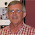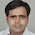## Friday, June 15, 2012

### F-tests Based on the HC or HAC Covariance Matrix Estimators

We all do it - we compute "robust" standard errors when estimating a regression model in any context where we suspect that the model's errors may be heteroskedastic and/or autocorrelated.

More correctly, we select the option in our favourite econometrics package so that the (asymptotic) covariance matrix for our estimated coefficients is estimated, using either White's heteroskedasticity-consistent (HC) estimator, or the Newey-West heteroskedasticity & autocorrelation-consistent (HAC) estimator.

The square roots of the diagonal elements of the estimated covariance matrix then provide us with the robust standard errors that we want. These standard errors are consistent estimates of the true standard deviations of the estimated coefficients, even if the errors are heteroskedastic (in White's case) or heteroskedastic and/or autocorrelated (in the Newey-West case).

That's fine, as long as we keep in mind that this is just an asymptotic result.

Then, we use the robust standard error to construct a "t-test"; or the estimated covariance matrix to construct an "F-test", or a Wald test.

And that's when the trouble starts!

So, what's the issue here?

My colleague, Graham Voss, and I were discussing this earlier this week.

To start with, the t-statistics won't be Student-t distributed in finite samples, under the null hypothesis, now that we've messed with the computation of the standard errors.

The square of a t-statistic with (n - k) degrees of freedom is usually F-distributed with 1 and (n - k) degrees of freedom.  (This post's title refers only to F-tests because of the usual equivalence between t2 and F statistics.) In our case, though, t2 won't be F-distributed any more.

The t-statistics will still have an asymptotic (null) distribution that's standard normal, as usual, if the null hypothesis is true.

Now, suppose that our F-statistic is computed for testing the validity of q independent linear restrictions, and that there are (n - k) degrees of freedom associated with the unrestricted model. Then, it would usually be the case that the finite-sample null distribution for our F-statistic is F, with q and (n - k) degrees of freedom. However, once we use the HC or HAC estimator of the covariance matrix, the F-statistic won't follow an F-distribution any more in finite samples, if the null is true.

Equally, it won't be non-central F-distributed under the alternative hypothesis. This has implications for the power of the test - it will no longer be UMPI.

What about the asymptotic (large n) case? Usually, qF converges in distribution to Chi Square with q degrees of freedom, under the null. It's then (asymptotically) equivalent to the Wald test. All of this will still hold if we're using the HC or HAC covariance estimator.

Alright, so this just tells us that if we're using the HC or HAC estimator, then if the sample is large enough we should treat the associated t-statistics as being standard normally distributed, if the null hypothesis is true. In addition, we should use the Wald test rather than the F-test, and the former's test statistic will have its usual Chi-Square distribution, under the null.

But wait, there's more!

Suppose that we have just a modest sized sample, and we don't think that we can realistically appeal to the asymptotics. What should we do? Specifically, should we use the t-statistics and F-statistics (based on the HC or HAC covariance matrix estimators), and "pretend" that the usual "t" and "F" null distributions apply? Or should we simply estimate the covariance matrix in the usual (inconsistent, if the errors are non-spherical) way, and calculate the "t" and "F" statistics as usual?

On the face of it, it's not clear which of these two "invalid" approaches will involve less distortion for the significance levels of the tests, and  have less impact on the tests' powers, in small samples.

In addition, we might ask, are there ways of modifying the t-tests and F-tests that are superior to the modifications implied by use of the HC or HAC covariance estimators?

Regrettably, this is not something that we see applied researchers taking into account very often. They just charge ahead with tests based on the HC or HAC estimators. That's a pity, because there's some pretty clear published evidence to guide us. And it's been around for a fairly long time!

Specifically, results established by Andrews (1991), Andrews and Monahan (1992), and Kiefer et al. (2000) indicate that:
•  F-tests and t-tests based on the HC or HAC covariance estimators generally exhibit substantial size distortion in finite samples.
• Typically, this distortion is upwards. So, you think that you're applying the test using a 5% significance level, say, but in reality the rate at which the null hypothesis is rejected (when it's true) may 10%, 20%, etc.
• The extent of this size distortion increases the greater the degree of heteroskedasticiy and/or autocorrelation in the model's errors.
• The test modifications suggested by Andrews and by Andrews and Monahan are quite effective in counteracting this size distortion.
• The modified test proposed by Kiefer et al. is generally superior to the other modified tests.

So, the next time that you opt for a HAC or HC estimator for the covariance matrix of  the estimator of the parameters in your model, just keep in mind that this may have major (negative) implications for your F-tests and t-tests, unless you have a very large sample.

And don't simply use a "canned" package without being aware of the relevant econometric theory. After all, there's no guarantee that the programmer had an appropriate level of awareness, is there?

References:

Andrews, D. W. K., 1991. Heteroskedasticity and autocorrelation consistent covariance matrix estimation. Econometrica, 59, 817- 858.

Andrews, D. W. K. and J. C. Monahan, 1992. An improved heteroskedasticity and autocorrelation consistent covaraince matrix estimator. Econometrica, 60, 953-966.

Kiefer, N. M., T. J. Vogelsang, and H. Bunzel, 2000. Simple robust testing of regression hypotheses. Econometrica, 68, 695-714.

© 2012, David E. Giles

#### 23 comments:

1.Or you could just use the bootstrap...

1.Absolutely!!!

2.I was at risk of making this mistake in writing a paper when a google search brought me to this post.

Just a question on the proposed bootstrap approach here. Which form of bootstrap are we talking about? Would a sieve bootstrap or block bootstrap work?

3.It would depend on the data you're using. If the data are time-series and i's autocorrelation that's the issue, then a block bootstrap would be a good choice.

4.Perfect!Thank you very much for the guidance.

2.Escanciano has a nice paper (though really theoretical ) about efficient testing methods for general semi-parametric models characterized by conditional moment restrictions.

http://mypage.iu.edu/~jescanci/FNPTApril272012.pdf

This is related to the post, as he shows that under conditional heteroskedasticity the t-test is not optimal (as we all know), but he proposes a test which is optimal.

The implementation of the procedure is not that complicated. Talking to him, he mention it would be maximum of 10 lines on matlab. Hoewever, I haven't tried yet.

=)

Guess this can be of interest of other readers.

1.Pedro: Thanks for that! Very helpful!

3.Continuing with the issue, Hausman and Palmery also has a paper on the poor performance of the t-ratio test under heteroskedasticity.

http://economics.mit.edu/files/7422

They propose a different test procedure based on Edgeworth expansions of the test statistic distribution.

1.Thanks again!

4.Hi,Giles!

That`s what Ed. Leamer calls White Washing, he also regrets this automatic use of robust standard erros. See his reply to Angrist and Pischke: http://www.anderson.ucla.edu/faculty/edward.leamer/selected_research/Tantalus%20by%20Leamer.pdf

Now, to your question: "In addition, we might ask, are there ways of modifying the t-tests and F-tests that are superior to the modifications implied by use of the HC or HAC covariance estimators?"

What about going back to model the heterokesdacity and doing some sensitivity anlysis? That is, to figure out how different assumptions in the heterokesdacity behaviou change our estimates and confidence intervals? We could also compare them with the robust confidence interval.

Thanks!

Carlos

1.Carlos : Right! The thing to keep in mind, though, that the modelling involved is unlikely to give exact inference in small samples.

5.In your final bullet point you refer to a test by Kiviet et al. Do you mean Kiefer?

6.Brian - thanks! Fixed.

7.HI Dave,
Thanks for your intersting post!
I was wondering whether, in practice, the issues raised in your post matter in a cross-section context as much as in a time-series context?
I have caught myself reiteratively using robust standard errors without giving it much thought. But on the other hand, I feel quite comfortable appealing to the CLT when working with a 1000 observations or more.

Best,
Boris

1.Boris - it's true that with microeconometric cross-section data, we often have thousands of observations, in which case the asymptotic results usually kick in (though sometimes surprisingly slowly).

Don't forget, though, that in macroecometrics we can be using cross section data with perhaps a hundered or so observations - e.g., one observation per country. Then, the finite sample issues are totally pertinent.

8.Dear Prof Giles,

A question on robust standard errors.

Since HETEROSCEDASTICTY makes OLS variances and SE larger, should we expect Robust S.E to be smaller than OLS SE?

But actually when we correct heteroscedasticty by White's Robust test, we find OLS SE can be larger or smaller than OLS SE.

Why it is so?

Kindly help.
Thank you.

1.The answer is that heteroskedasticity DOESN'T necessarily make the OLS standard errors larger. They can be larger or smaller than they would otherwise be. Which way they are distorted will depend on the the relationship between the (changing) variance of the errors, and the pattern of the variability of the regressors in the sample. Likewise, the het-consistent standard errors can be larger or smaller than the regular standard errors in the face of heteroskedasticity.

9.Dear Prof Giles,

Would this also apply to the cluster-robust variance matrix estimator ? [I think it is vce(cluster) in stata]
Or is there another modification that would be required to do an F-test?

Thanks

Louis

1.Yes it would. (I don't use Stata.)

10.Sir, can we use White test for heteroscedasticity, when there are quantitative and dummy regressors in the OLS regression? Please guide

1.Varun - yes you can. But the comments in this post will still apply.

2.Thanks for the reply Sir, i had a query as someone told me that when we have dummies the white test does not work. And I did not find of anything of that sort in literature. That someone had read in paper, which he cant recollect.

3.I'm sure you know that White's test uses the regressors, their squares, and cross-products inan "auxilliary" regression to form he test statistic. Squaring a dummy variable produces just the same dummy variable. Obviously you don't try and include the dummy and its square in the auxilliary regression. This is a standard feature of the test - for any regressors. EViews, for instance checks if this is an issue and drops any perfectly collinear regresors from the auxilliary regression.